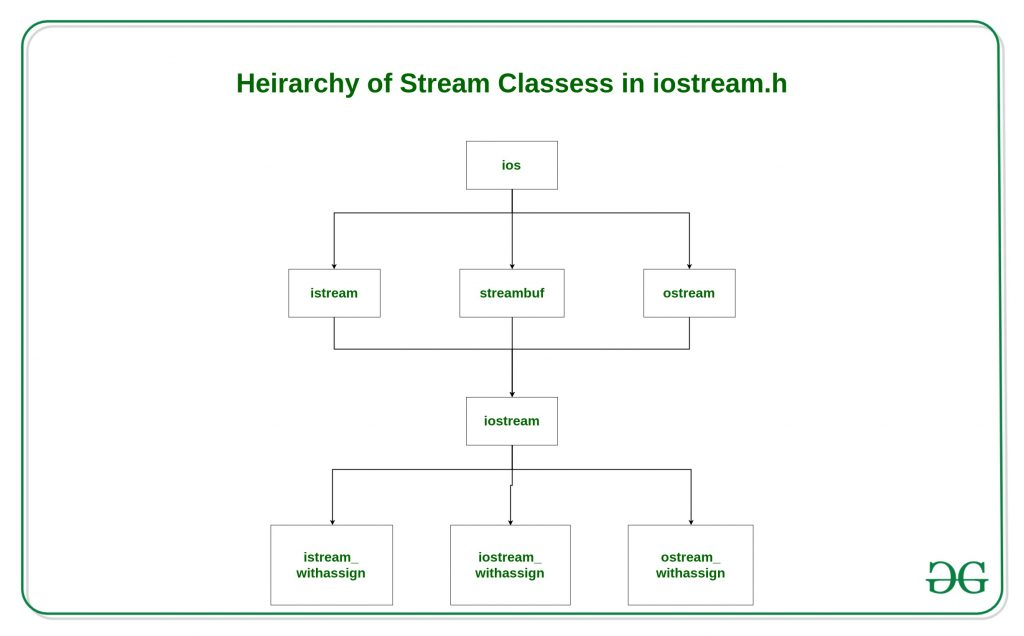Related Articles

# C++ Stream Classes Structure

• Difficulty Level : Hard
• Last Updated : 24 Jun, 2021

In C++ there are number of stream classes for defining various streams related with files and for doing input-output operations. All these classes are defined in the file iostream.h. Figure given below shows the hierarchy of these classes.1. ios class is topmost class in the stream classes hierarchy. It is the base class for istream, ostream, and streambuf class.

2. istream and ostream serves the base classes for iostream class. The class istream is used for input and ostream for the output.

3. Class ios is indirectly inherited to iostream class using istream and ostream. To avoid the duplicity of data and member functions of ios class, it is declared as virtual base class when inheriting in istream and ostream as

```class istream: virtual public ios
{
};
class ostream: virtual public ios
{
};```

The _withassign classes are provided with extra functionality for the assignment operations that’s why _withassign classes.

Facilities provided by these stream classes.

1. The ios class: The ios class is responsible for providing all input and output facilities to all other stream classes.

2. The istream class: This class is responsible for handling input stream. It provides number of function for handling chars, strings and objects such as get, getline, read, ignore, putback etc..
Example:

## CPP14

 `#include ``using` `namespace` `std;` `int` `main()``{``    ``char` `x;` `    ``// used to scan a single char``    ``cin.get(x);` `    ``cout << x;``}`

Input:

`g`

Output:

`g`

The ostream class: This class is responsible for handling output stream. It provides number of function for handling chars, strings and objects such as write, put etc..
Example:

## CPP14

 `#include ``using` `namespace` `std;` `int` `main()``{``    ``char` `x;` `    ``// used to scan a single char``    ``cin.get(x);` `    ``// used to put a single char onto the screen.``    ``cout.put(x);``}`
1. Input:

`g`

Output:

`g`

The iostream: This class is responsible for handling both input and output stream as both istream class and ostream class is inherited into it. It provides function of both istream class and ostream class for handling chars, strings and objects such as get, getline, read, ignore, putback, put, write etc..
Example:

## CPP14

 `#include ``using` `namespace` `std;` `int` `main()``{` `    ``// this function display``    ``// ncount character from array``    ``cout.write(``"geeksforgeeks"``, 5);``}`

Output:

`geeks`

istream_withassign class: This class is variant of istream that allows object assignment. The predefined object cin is an object of this class and thus may be reassigned at run time to a different istream object.
Example:To show that cin is object of istream class.

## CPP14

 `#include ``using` `namespace` `std;` `class` `demo {``public``:``    ``int` `dx, dy;` `    ``// operator overloading using friend function``    ``friend` `void` `operator>>(demo& d, istream& mycin)``    ``{``        ``// cin assigned to another object mycin``        ``mycin >> d.dx >> d.dy;``    ``}``};` `int` `main()``{``    ``demo d;``    ``cout << ``"Enter two numbers dx and dy\n"``;` `    ``// calls operator >> function and``    ``// pass d and cin as reference``    ``d >> cin; ``// can also be written as operator >> (d, cin) ;` `    ``cout << ``"dx = "` `<< d.dx << ``"\tdy = "` `<< d.dy;``}`

Input:

`4 5`

Output:

```Enter two numbers dx and dy
4 5
dx = 4  dy = 5```

ostream_withassign class: This class is variant of ostream that allows object assignment. The predefined objects cout, cerr, clog are objects of this class and thus may be reassigned at run time to a different ostream object.
Example:To show that cout is object of ostream class.

## CPP14

 `#include ``using` `namespace` `std;` `class` `demo {``public``:``    ``int` `dx, dy;` `    ``demo()``    ``{``        ``dx = 4;``        ``dy = 5;``    ``}` `    ``// operator overloading using friend function``    ``friend` `void` `operator<<(demo& d, ostream& mycout)``    ``{``        ``// cout assigned to another object mycout``        ``mycout << ``"Value of dx and dy are \n"``;``        ``mycout << d.dx << ``" "` `<< d.dy;``    ``}``};` `int` `main()``{``    ``demo d; ``// default constructor is called` `    ``// calls operator << function and``    ``// pass d and cout as reference``    ``d << cout; ``// can also be written as operator << (d, cout) ;``}`

Output:

```Value of dx and dy are
4 5```

Related Articles

Want to learn from the best curated videos and practice problems, check out the C++ Foundation Course for Basic to Advanced C++ and C++ STL Course for foundation plus STL.  To complete your preparation from learning a language to DS Algo and many more,  please refer Complete Interview Preparation Course.

My Personal Notes arrow_drop_up